On Growth and Form 2.0 Midterm Project
Chloe Chao
Spring 1997

The Klein bottle is a closed surface that has no interior. Perhaps its closest kin is the Mobius strip. Regardless, the basic principle of continuity is the same.

In the following images (which you can click on to make larger), I have used the parametric equations defined below to define a Klein bottle that is two Mobius strips joined together to make a closed surface.

Here is a quicktime movie of one of my klein examples.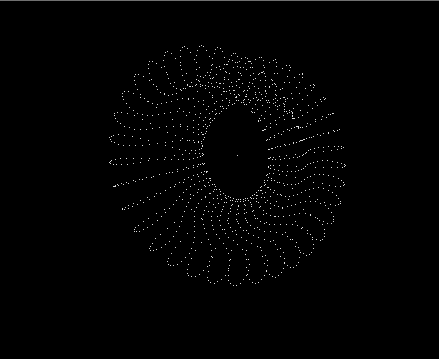This first image is the points generated by the parametric equations: x = cos(u)*(a+(sin(v)*cos(u/2.0))-((sin(v*2.0)*sin(u/2.0)/2.0))) y = sin(u)*(a+(sin(v)*cos(u/2.0))-((sin(v*2.0)*sin(u/2.0)/2.0))) z = (sin(u/2.0)*sin(v))+(cos(u/2.0)*sin(v*2.0)/2.0) where u and v are angles ranging from -pi to pi.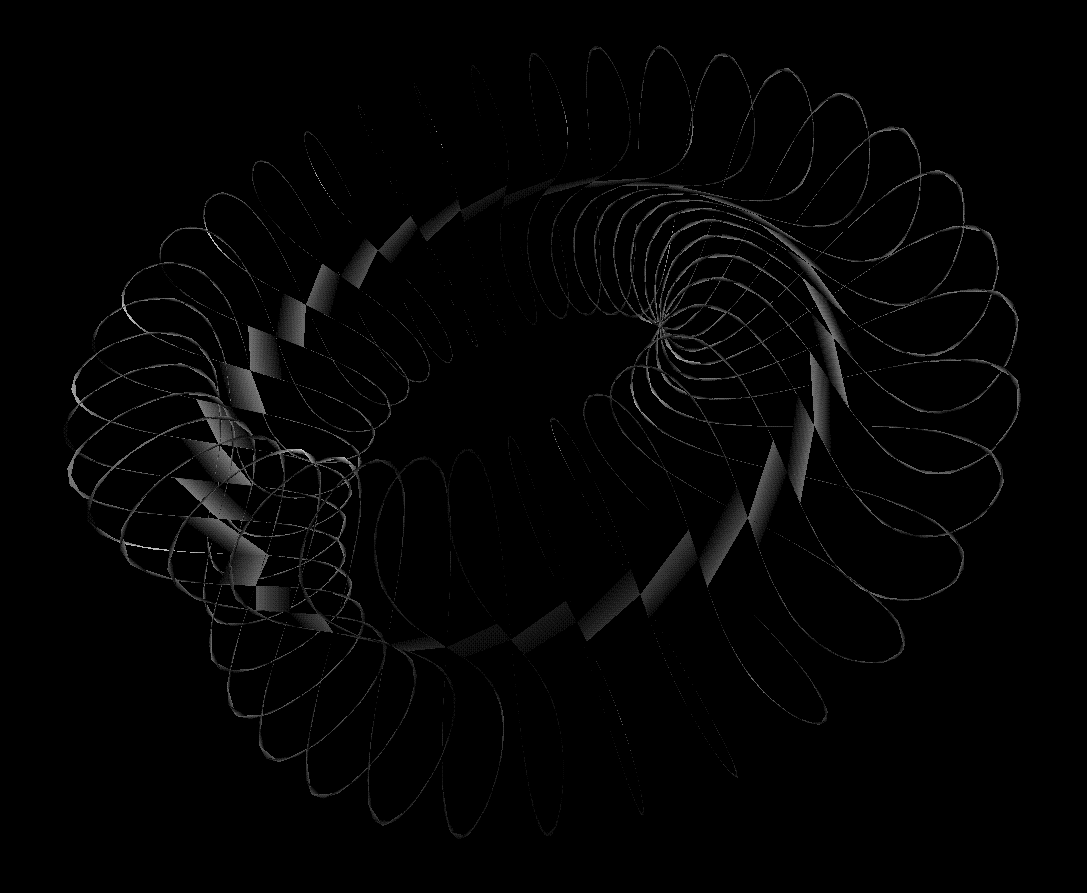This second image is the same set of points with triangular strips drawn between adjacent points.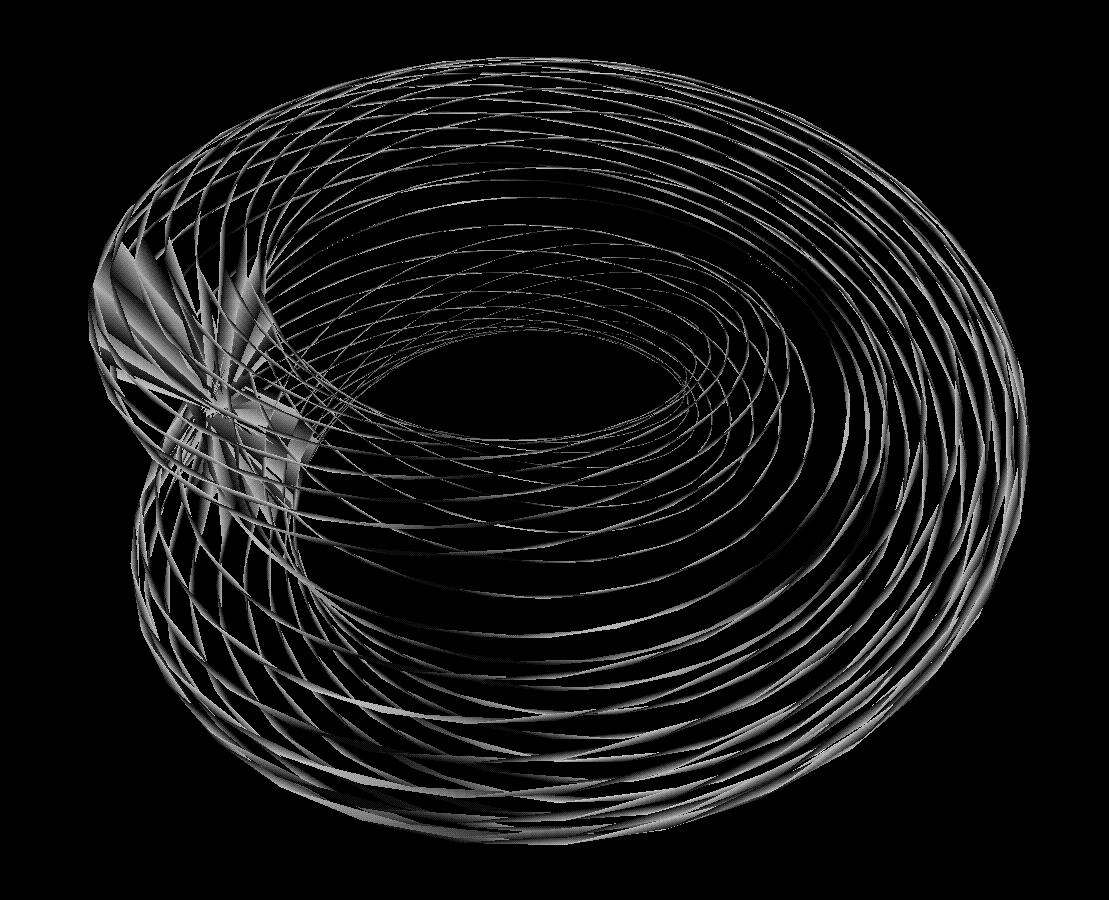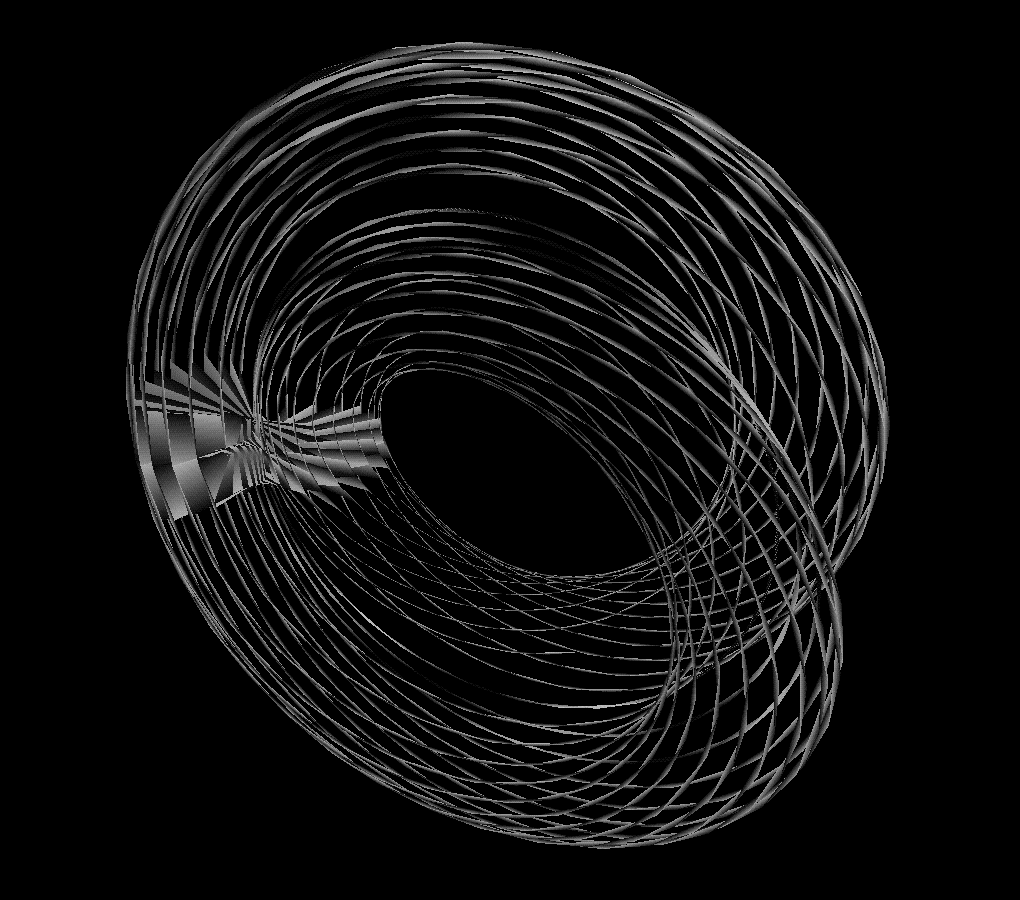These next images are the same set of points, but with triangular strips drawn between the points in a different order. This is my favorite representation of the form in that the ribbon-like quality of the surface fills out the form and empasizes the relationship of the form to the Mobius strip.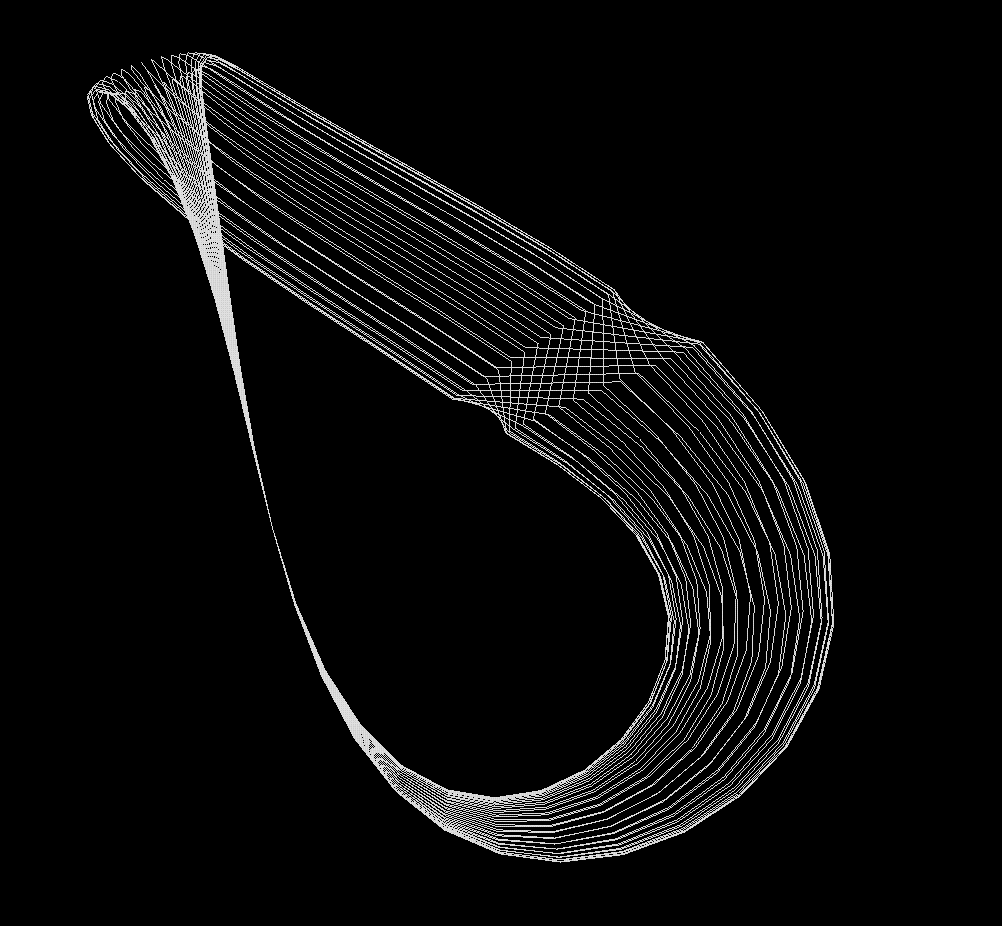This last image is a more traditional representation of the Klein bottle. The points are generated by the parametric equations: when u is less than pi x = (6*cos(a)*(1+sin(a)))+(r*cos(a)*cos(b)) y = (16*sin(a))+(r*sin(a)*cos(b)) when u is greater than pi x = (6*cos(a)*(1+sin(a)))+(r*cos(b+180)) y = (16*sin(a)) z = r*sin(b) where u and v are angles ranging from 0 to 2pi.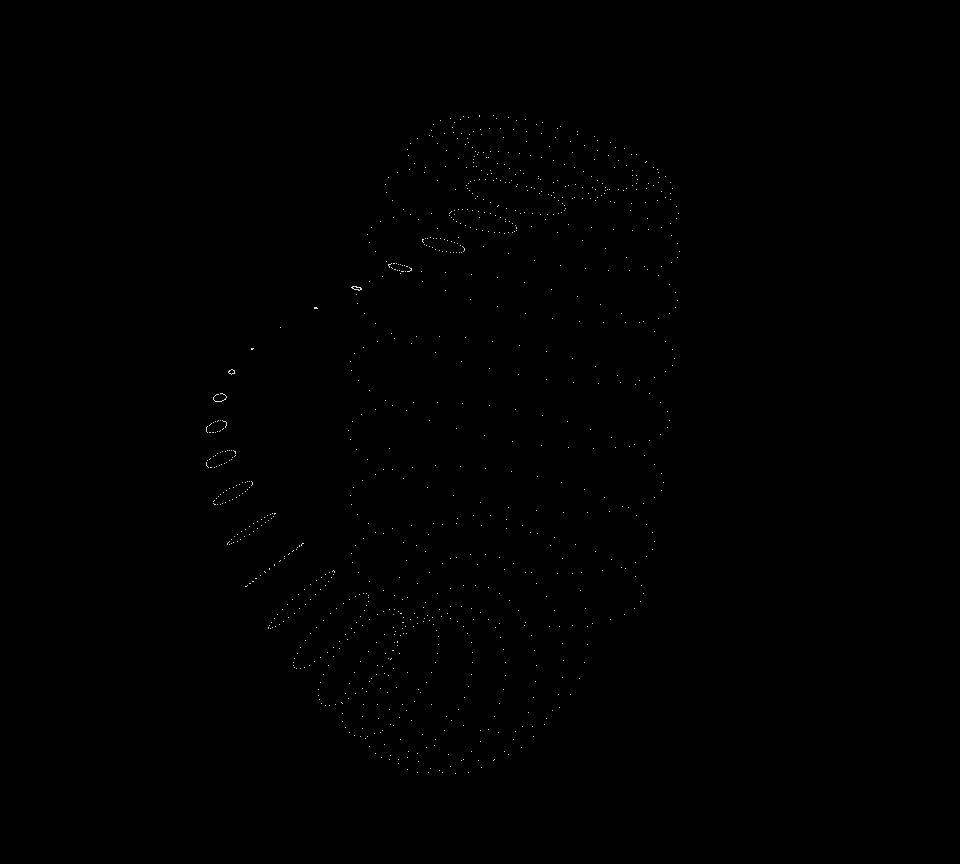We can better understand the form by viewing the points that it is composed of. In this image to the left we can observe how it appears that the bottom of the bottle stretches back up to intersect the neck of the bottle and become the mouth of the bottle.

To realize the Klein bottles in 3D requires the surface to intersect itself. What I would be interested in is to perhaps define the Klein bottle over time, thereby adding a "fourth" dimension.

Methods: The program that generates the points is written in C++ and uses the Inventor C++ graphics libraries to display the point/line sets to the screen.

Acknowledgements/References: The parametric equations for these forms can be found at Paul Bourke's site, which in general is an excellent computer graphics reference site.